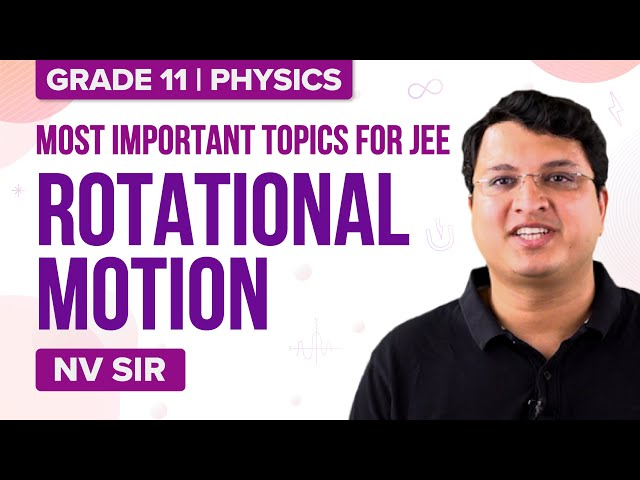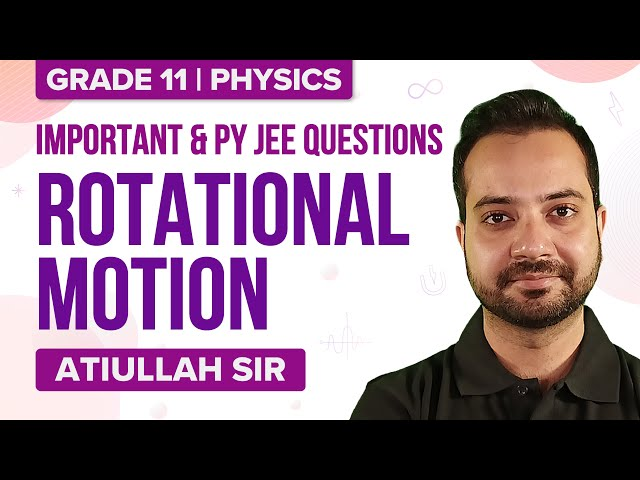Checkout JEE MAINS 2022 Question Paper Analysis : Checkout JEE MAINS 2022 Question Paper Analysis :

# JEE Advanced Previous Year Questions with Solutions on Rotational Dynamics

In a circular or rotatory motion, a body may rotate about an axis passing through itself or about an axis outside. Different particles of the body will move with different velocities, the velocity of a particle is directly proportional to its distance from the axis of rotation. Therefore the particle cannot be treated as a single particle as in translatory motion. The shape and size of the body have to be considered.

Question 1) A circular plate of uniform thickness has a diameter of 56 cm. A circular portion of diameter 42 cm is removed from one edge of the plate as shown in the figure. Find the position of the centre of mass of the remaining portion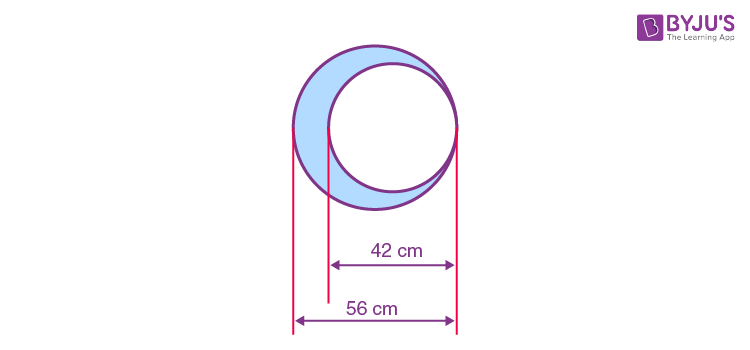Answer: Centre of mass lies at a distance of 9 cm from the origin towards left

Solution: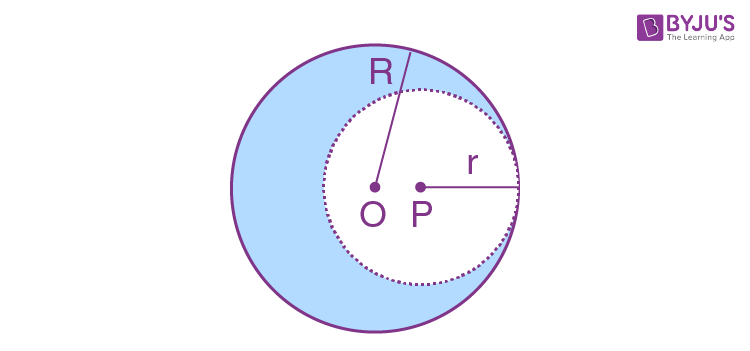Let σ be the mass per unit area of the uniform plate

Mass of the whole disc = σ x πR2

Mass of the portion removed = σ x πr2

R = 28 cm, r = 21 cm, OP = 7 cm

Position of the centre of mass

$$\begin{array}{l}x = \frac{m_{1}x_{1}-m_{2}x_{2}}{m_{1}-m_{2}}\end{array}$$
$$\begin{array}{l}x = \frac{\sigma \times \pi R^{2(0)}-\sigma \times \pi r^{2}\times 7}{\sigma \pi R^{2}-\sigma \pi r^{2}}\end{array}$$
$$\begin{array}{l}x = \frac{-(21)^{2}\times 7}{(28)^{2}-(21)^{2}}= -9 cm\end{array}$$

The centre of mass lies at a distance of 9 cm from the origin towards left

Question 2) A solid sphere of mass 2 kg radius 0.5m is rolling with an initial speed of 1 ms-1 goes up an inclined plane which makes an angle of 300 with the horizontal plane, without slipping. How long will the sphere take to return to the starting point A?(A) 0.80 s

(B) 0.60 s

(C) 0.52 s

(D) 0.57 s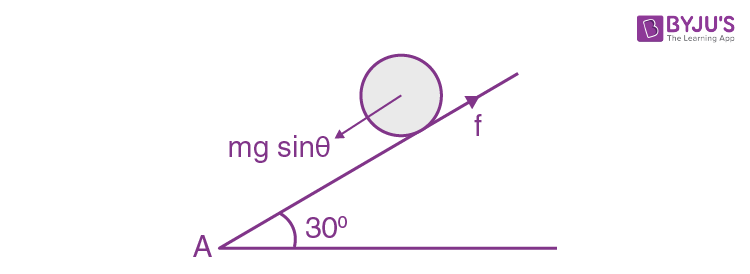$$\begin{array}{l}a = \frac{gsin\theta }{1+c}\end{array}$$

For a solid sphere, c = ⅖

$$\begin{array}{l}a = \frac{9.8 sin 30^{0} }{1+\frac{2}{5}}\end{array}$$

a = 3.5 m/sec2

Time of ascent is given by

v = u + at

0 = 1 – 3.5 t

$$\begin{array}{l}t= \frac{1}{3.5} sec\end{array}$$

Time of decent

$$\begin{array}{l}t= \frac{1}{3.5} sec\end{array}$$
(due to symmetry of motion)

Total time,

$$\begin{array}{l}T= \frac{2}{3.5} sec\end{array}$$
= 0.57 sec

Question 3) When a disc slides on a smooth inclined surface from rest, the time taken to move from A to B is t1. When the disc performs pure rolling from rest then the time taken to move from A to B is t2. If

$$\begin{array}{l}\frac{t_{1}}{t_{2}}=\sqrt{\frac{3}{x}}\end{array}$$
find x.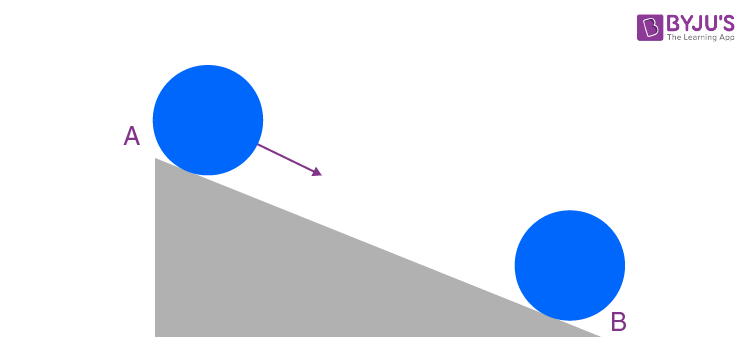(A) 2

(B) 1

(C) 5

(D) 7

Solution:

When disc slides a1 = gsinθ

So,

$$\begin{array}{l}S = ut_{1}+\frac{1}{2}a_{1}t_{1}^{2}=\frac{1}{2}gsin\theta t_{1}^{2}\end{array}$$
…………(1)

When disc do pure rolling

$$\begin{array}{l}a_{2}=\frac{gsin\theta }{1+\frac{k^{2}}{R^{2}}}=\frac{gsin\theta }{1+1/2}=\frac{2}{3}gsin\theta\end{array}$$

So,

$$\begin{array}{l}S= ut_{2}+\frac{1}{2}a_{2}t_{2}^{2}=\frac{1}{2}\times \frac{2}{3}gsin\theta t_{2}^{2}\end{array}$$
——-(2)

From (1) and (2)

$$\begin{array}{l}\frac{t_{2}}{t_{1}}=\sqrt{\frac{3}{2}}\end{array}$$

So, x = 2

Question 4) A wheel rotating with an angular speed of 600 rpm is given a constant acceleration of 1800 rpm2 for 10 sec. The number of revolutions revolved by the wheel is

(A) 125

(B) 100

(C) 75

(D) 50

Solution: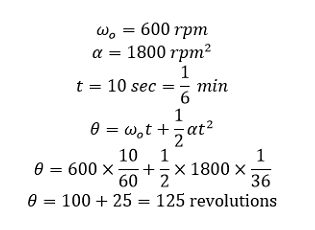Question 5) For a body in pure rolling, its rotational kinetic energy is 1/2 times of its translational kinetic energy. The body should be?

(A) Solid cylinder

(B) Ring

(C) Solid sphere

(D) Hollow sphere

Solution:

Given,

Rotational K.E = (½) Translational K.E

(½)Iω2 = (½) x (½)mv2

In pure rolling, v = Rω

(½)Iω2 = (1/4) mR2ω2

I = (½) mR2

Here, it is a solid cylinder

Question 6) Four solid spheres each of diameter √5 cm and mass 0.5 kg are placed with their centres at the corners of a square of side 4 cm. The moment of inertia of the system about the diagonal of the square is N x 10-4 kgm2, then N is

Solution: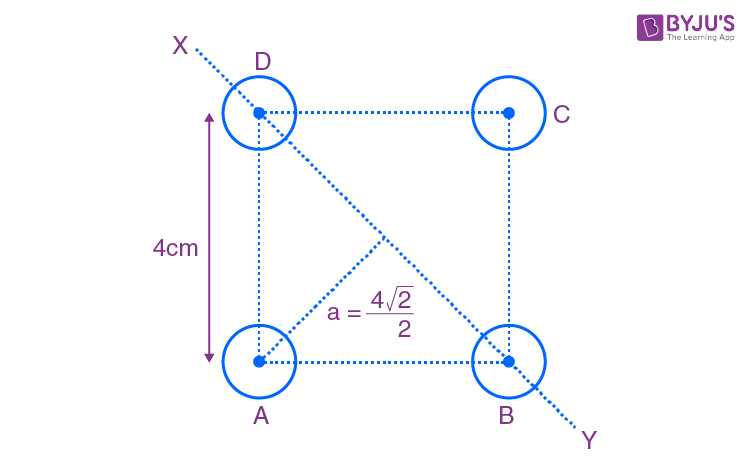Ixy = IA + IB + IC+ID

By symmetry

IA = IC

IB = ID

Ixy = 2IA +2 IB

$$\begin{array}{l}I_{xy}= 2\left [ \frac{2}{5}MR^{2}+Ma^{2} \right ]+2\left [ \frac{2}{5}MR^{2}\right ]\end{array}$$
$$\begin{array}{l}I_{xy}= 4\times \frac{2}{5}MR^{2}+2Ma^{2}\end{array}$$
$$\begin{array}{l}I_{xy}= M\times \left [ \frac{8}{5}MR^{2}+2Ma^{2} \right ]\end{array}$$
$$\begin{array}{l}I_{xy}= \left [ \frac{8}{5}\times 0.5\times (\frac{\sqrt{5}}{2})^{2}+2\times (0.5)\times (4\times 2)^{2} \right ]\times 10^{-4}\end{array}$$

= 9 x 10-4

= N x 10-4

Therefore, N = 9

Question 7) A boy is pushing a ring of mass 2 kg and radius 0.5 m with a stick as shown in the figure. The stick applies a force of 2N on the ring and rolls it without slipping with an acceleration of 0.3 m/s2. The coefficient of friction between the ground and the ring is large enough that rolling always occurs and the coefficient of friction between the stick and the ring is (P/10). The value of P is

(A) 1

(B) 2

(C) 3

(4) 4

Solution:

The stick applies (2N) force so the point of contact O of the ring with ground tends to slide. But the frictional force f2 does not allow this and creates a torque about ‘c, which starts rolling the ring. Between the ring and the stick, a frictional force f1 also acts.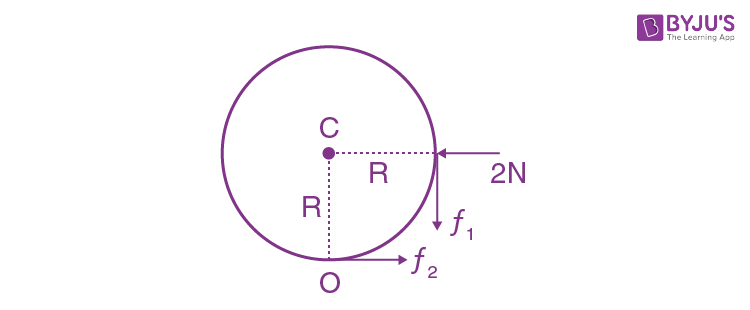F – f2 = ma

2 – f2 = 2 x 0.3

Therefore, f2 = 1.4 N

Applying τ = Iα about C

(f2 – f1) R = Iα = I(a/R)

(1.4 – μ x 2) x 0.5 = 2 x (0.5)2 x (0.3/0.5) (since I = MR2]

μ= 0.4 = 4/10 = P/10

Therefore, P = 4

Question 8) A roller is made by joining together two cones at their vertices O. It is kept on two rails AB and CD, which are placed asymmetrically (see figure), with its axis perpendicular to CD and its centre O at the centre of line joining AB and CD. It is given a light push so that it starts rolling with its centre O moving parallel to CD in the direction shown. As it moves, the roller will tend to: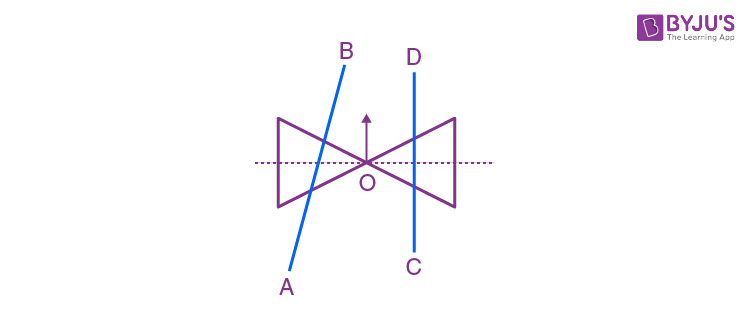(A) go straight

(B) Turn left and right alternately

(C) Turn left

(D) Turn right

Solution: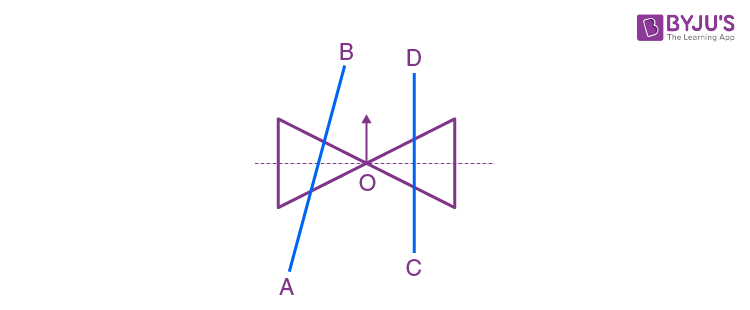As shown in the diagram, the normal reaction of AB on the roller will shift towards O

This will lead to the tending of the system of cones to turn left.

Question 9) A loop of radius r and mass m rotating with an angular velocity ω0 is placed on a rough horizontal surface. The initial velocity of the centre of the hoop is zero. What will be the velocity of the centre of the hoop when it ceases to slip?

(A) rω0/4

(B) rω0/3

(C) rω0/2

(D) rω0

Solution:

From the conservation of angular momentum about any fix point on the surface,

mr2ω0 = 2mr2ω

⇒ ω = ω0/2

⇒ v =(ω0/2)r

Question 10) A homogeneous solid cylindrical roller of radius R and mass M is pulled on a cricket pitch by a horizontal force. Assuming rolling without slipping, angular acceleration of the cylinder is:

(A) 3F/2mR

(B) F/3mR

(C) F/2mR

(D) 2F/3mR

Solution: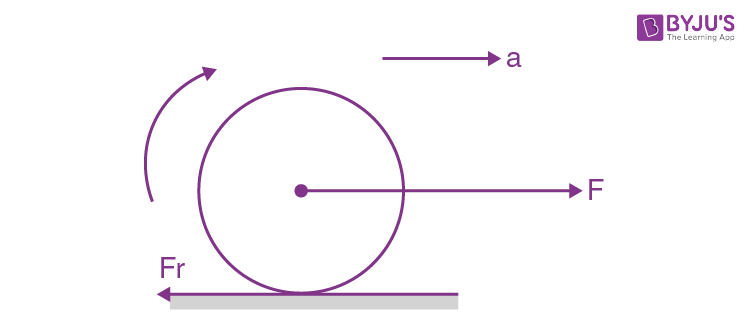F- fr = ma ——-(1)

frR = Iα = (mR2/2)α ——(2)

For pure rolling

a = αR ———(3)

From (1), (2) and (3)

F – (mRα/2) = mαR

⇒ α = 2F/3mR